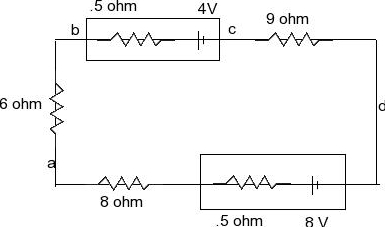# Problem: a) What is the potential difference Vad in thecircuit?b) What is the terminal voltage of the 4 V battery?c) A battery with emf 10.3 V and internal resistance .5ohm isinserted in the circuit d with its negative terminal connected tothe negative terminal of the 8 V battery. WHat is the difference ofpotential Vbc between the terminals of the 4 V batterynow?

###### FREE Expert Solution

Kirchhoff's loop rule:

and

Voltage,

V = iR

Let's apply the Kirchhoff's loop rule starting with the 8V battery going in the clockwise direction.

8 - i0.5 - i8 - i6 - i0.5 - 4 - i9 = 0

- i24 = - 8

i = 1/6 A

a)

Starting from point a to point b, we have:

Va + i8 + i0.5 - 8 - Vd = 0

91% (337 ratings)###### Problem Detailsa) What is the potential difference Vad in thecircuit?

b) What is the terminal voltage of the 4 V battery?

c) A battery with emf 10.3 V and internal resistance .5ohm isinserted in the circuit d with its negative terminal connected tothe negative terminal of the 8 V battery. WHat is the difference ofpotential Vbc between the terminals of the 4 V batterynow?